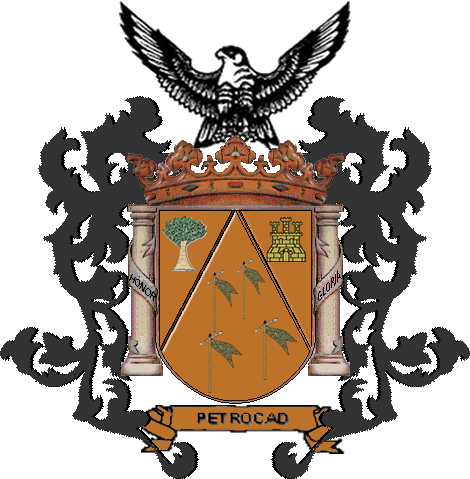Acticles

STORAGE TANK UNITS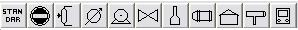All this information could be changed suddenly
•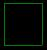Tank. Choose this store unit when you want to store your product to open vessel. When you drag this unit to the main screenwork, that appear.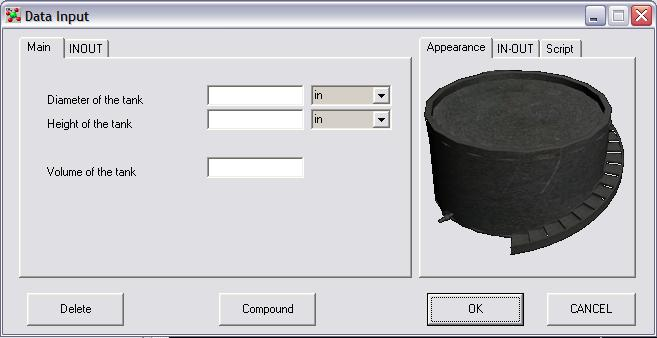Set the diameter of tank(D), set the height of the tank(H), The Volume will be computed in this way.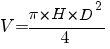Now, select the INOUT TAB. That appear.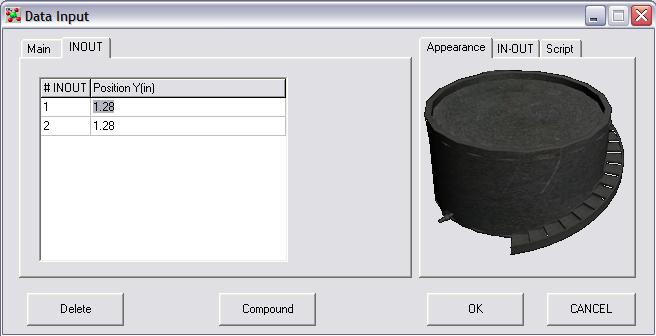Each # INOUT called Hn, correspond to each In or Out of the tank, called take. This number is the height(Hn) of each take. By default each take is 12.8% of height of the tank.This number is used to compute the pressure in each take. The Pressure is computed in this way :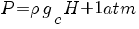where H = Level of liquid - Hn In Each iteraction, the pressure of all take are computed, the temperature of the tank is computed, also the composition could be computed if it was necessary.
•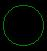Sphere. Choose this storage unit when you want to store your products to closed vessel. When you drag this unit to the main screenwork, that appear.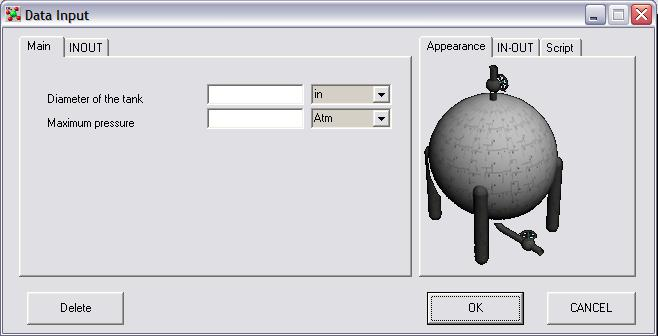Set the diameter of the tank(D), and set maximum pressure, The volume will be computed in this way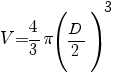Now, select the INOUT TAB. That appear.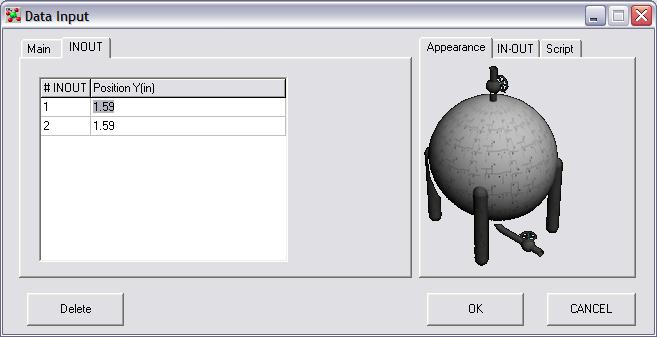Each # INOUT called Hn, correspond to each In or Out of the tank, called take. This number is the height(Hn) of each take. By default each take is 15.9% of the diameter of the tank. This number is used to compute the pressure in each take. The Pressure is computed in this way :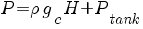where H = Level of liquid - Hn & PTANK = Pressure of tank in this moment. This value change because the volume of gas into the sphere change.